# 1.1   算法无处不在¶

1. 翻开字典约一半的页数，查看该页的首字母是什么，假设首字母为 $$m$$
2. 由于在拼音字母表中 $$r$$ 位于 $$m$$ 之后，所以排除字典前半部分，查找范围缩小到后半部分。
3. 不断重复步骤 1. 和 步骤 2. ，直至找到拼音首字母为 $$r$$ 的页码为止。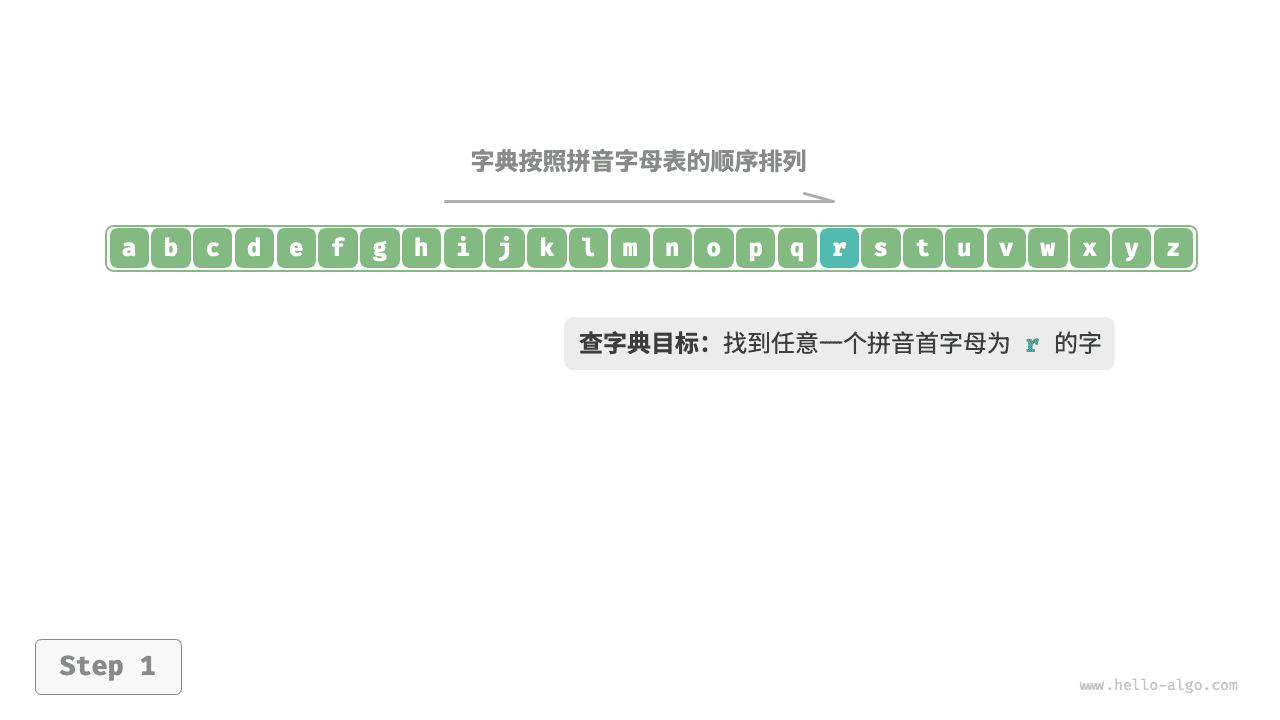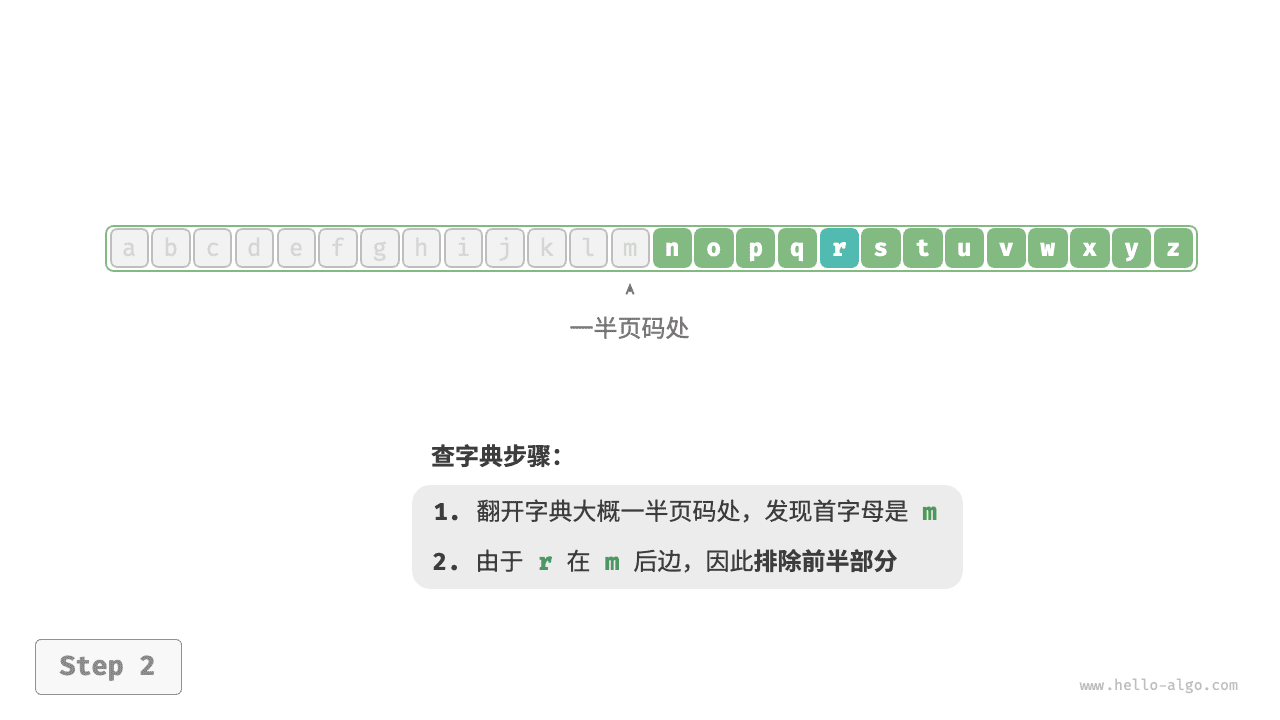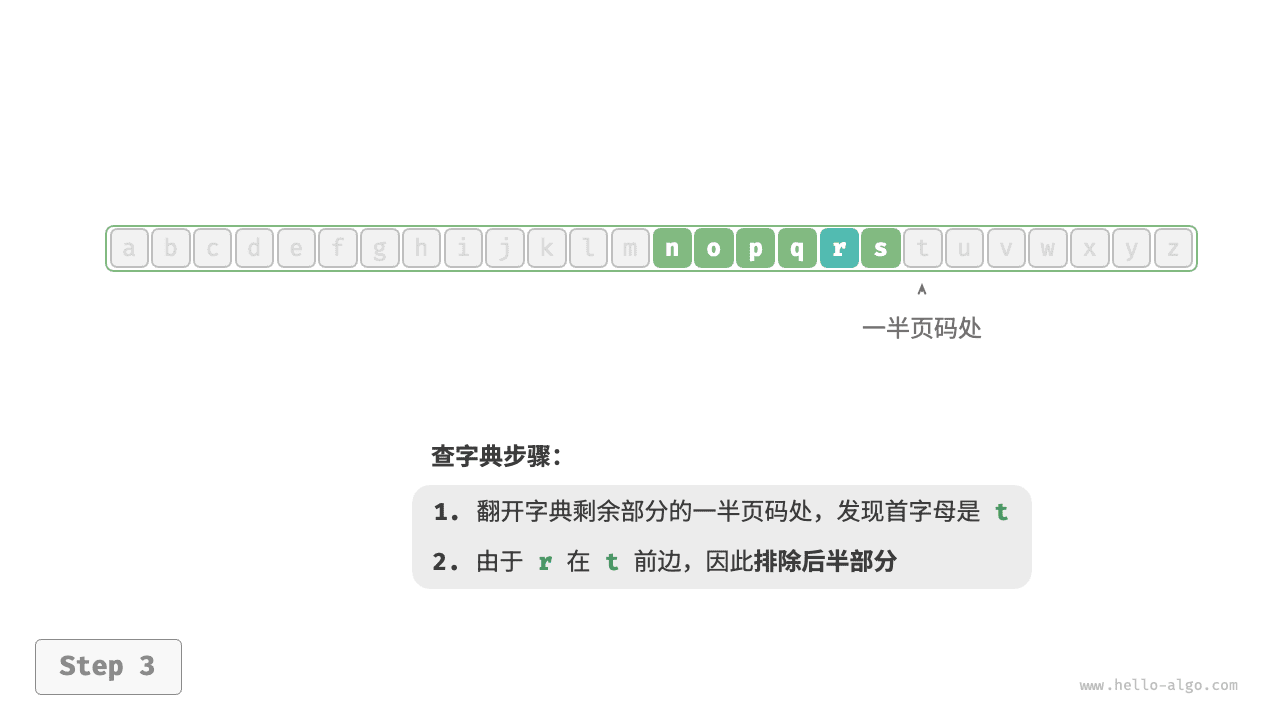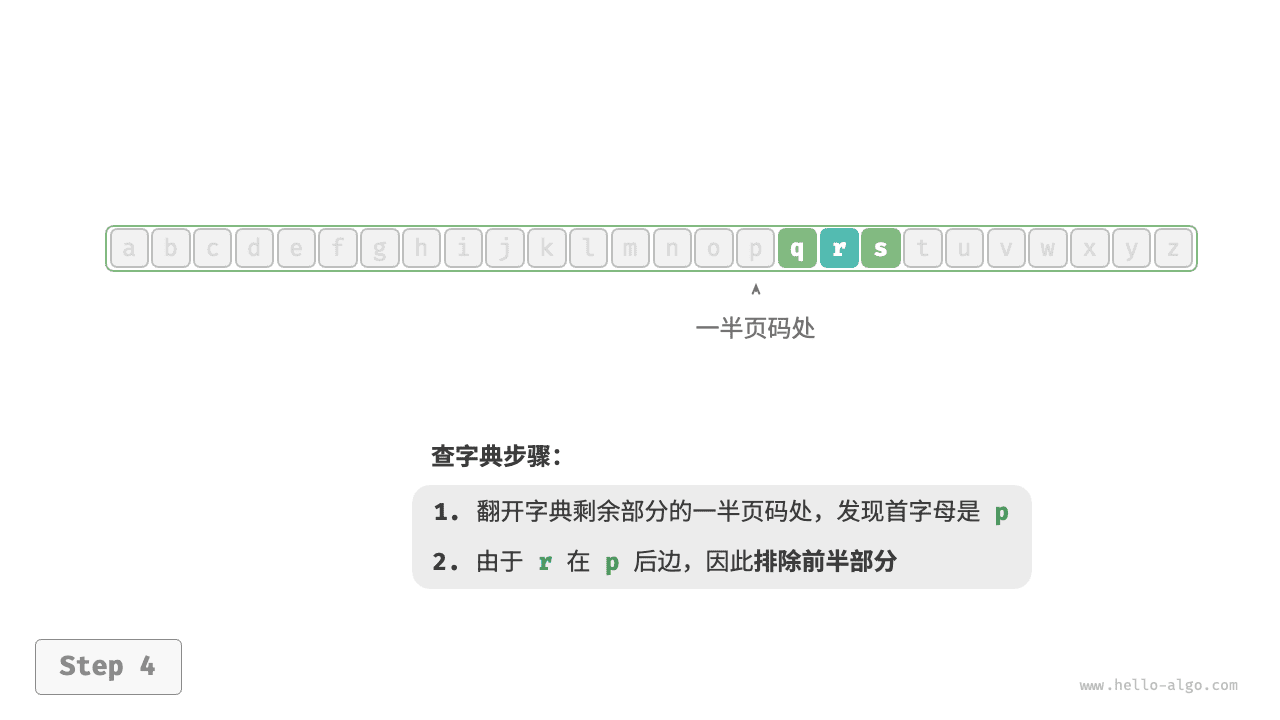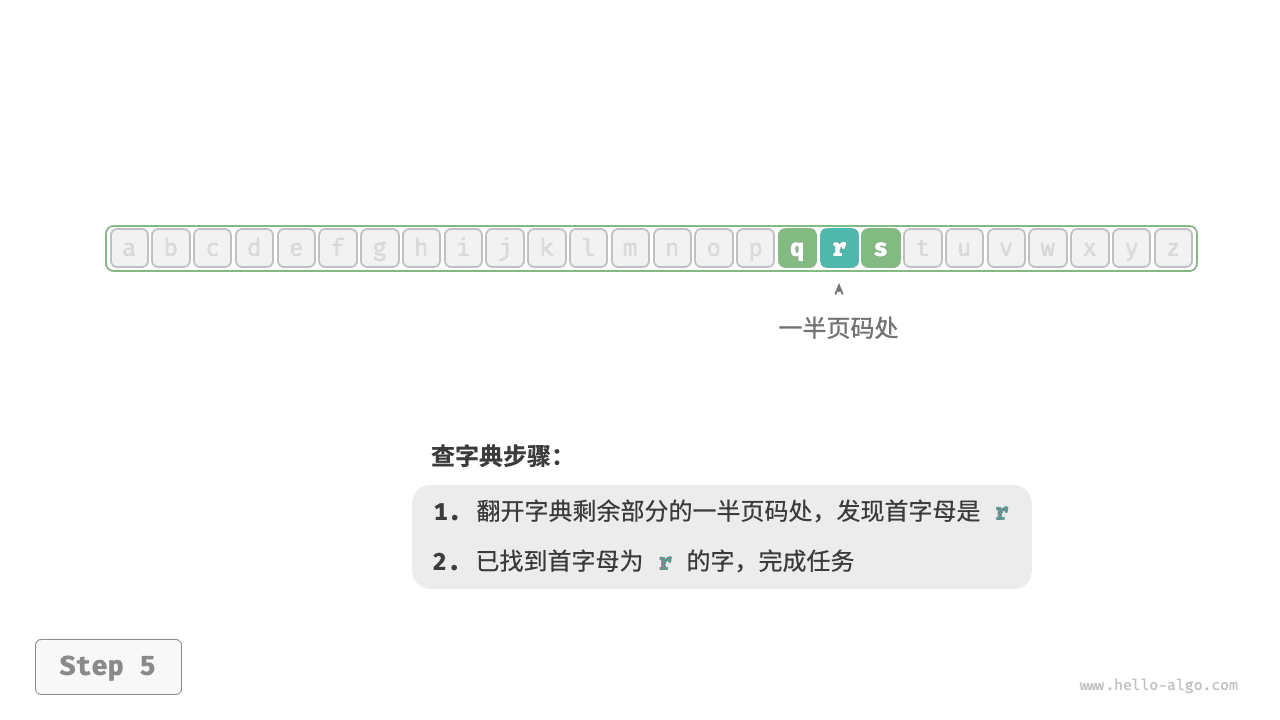1. 将扑克牌划分为“有序”和“无序”两部分，并假设初始状态下最左 1 张扑克牌已经有序。
2. 在无序部分抽出一张扑克牌，插入至有序部分的正确位置；完成后最左 2 张扑克已经有序。
3. 不断循环步骤 2. ，每一轮将一张扑克牌从无序部分插入至有序部分，直至所有扑克牌都有序。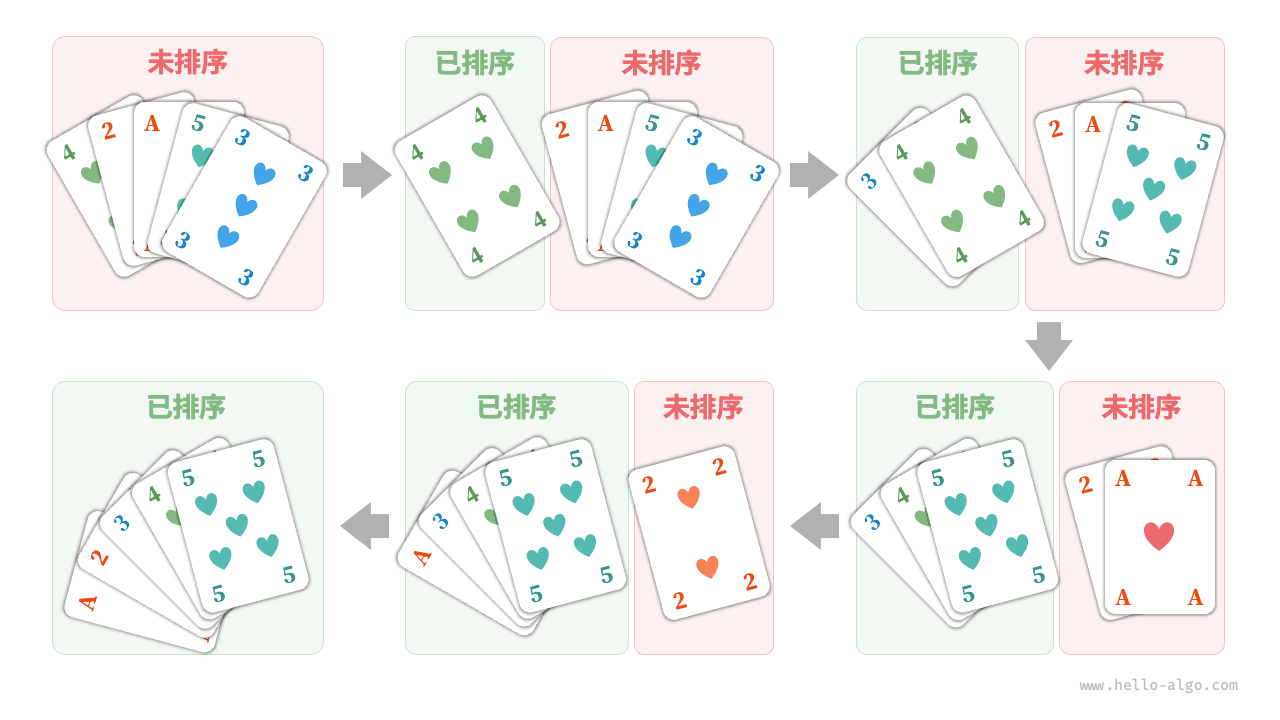1. 可选项是比 $$31$$ 元面值更小的货币，包括 $$1$$ 元、$$5$$ 元、$$10$$ 元、$$20$$ 元。
2. 从可选项中拿出最大的 $$20$$ 元，剩余 $$31 - 20 = 11$$ 元。
3. 从剩余可选项中拿出最大的 $$10$$ 元，剩余 $$11 - 10 = 1$$ 元。
4. 从剩余可选项中拿出最大的 $$1$$ 元，剩余 $$1 - 1 = 0$$ 元。
5. 完成找零，方案为 $$20 + 10 + 1 = 31$$ 元。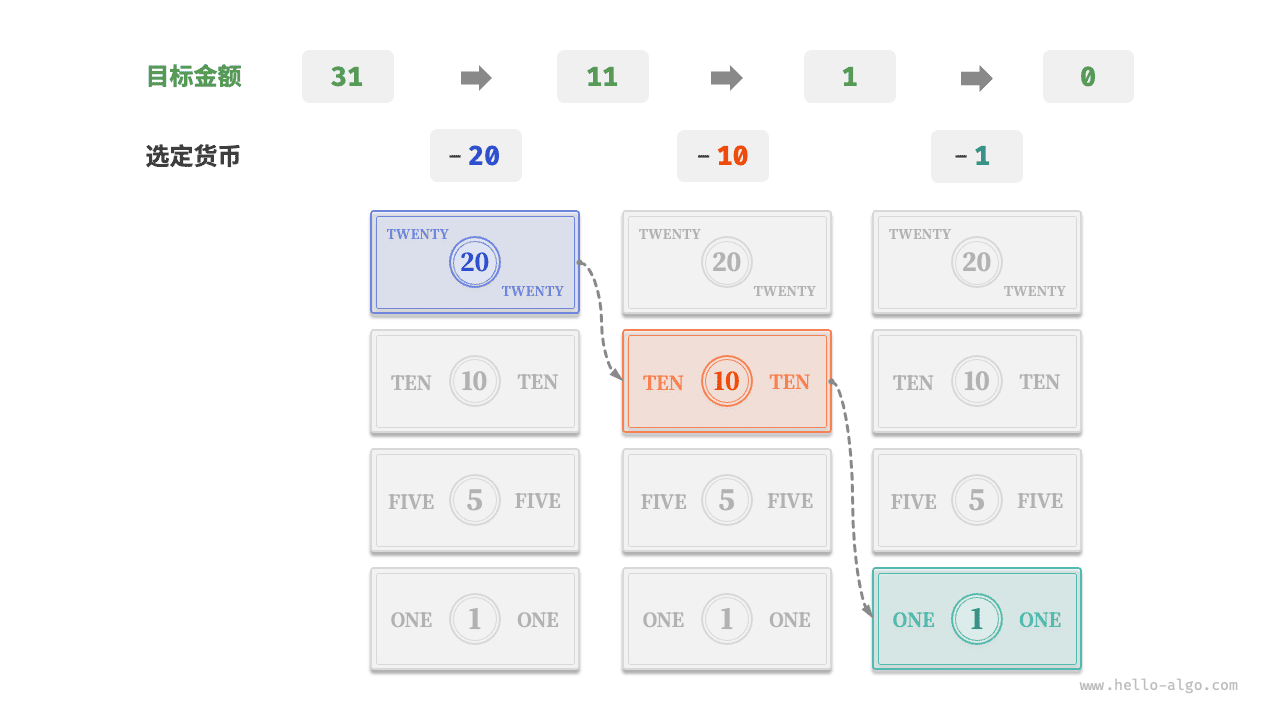Tip